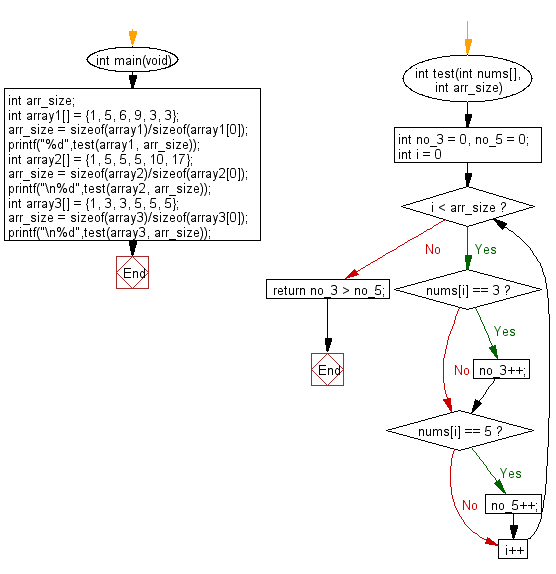﻿ C exercises: Check if the number of 3's is greater than the number of 5's - w3resource# C Exercises: Check if the number of 3's is greater than the number of 5's

## C-programming basic algorithm: Exercise-57 with Solution

Write a C program to check if the number of 3's is greater than the number of 5's.

C Code:

``````#include <stdio.h>
#include <stdlib.h>

int main(void){
int arr_size;
int array1[] = {1, 5, 6, 9, 3, 3};
arr_size = sizeof(array1)/sizeof(array1);
printf("%d",test(array1, arr_size));
int array2[] = {1, 5, 5, 5, 10, 17};
arr_size = sizeof(array2)/sizeof(array2);
printf("\n%d",test(array2, arr_size));
int array3[] = {1, 3, 3, 5, 5, 5};
arr_size = sizeof(array3)/sizeof(array3);
printf("\n%d",test(array3, arr_size));
}

int test(int nums[], int arr_size)
{
int no_3 = 0, no_5 = 0;

for (int i = 0; i < arr_size; i++)
{
if (nums[i] == 3) no_3++;
if (nums[i] == 5) no_5++;
}

return no_3 > no_5;
}
``````

Sample Output:

```1
0
0
```

Pictorial Presentation:Flowchart:C Programming Code Editor: